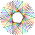CipherJan 7, 2017

William Herschel - Uranus and Moons of SaturnWilliam Herschel = 157 (37th prime number), died at 'Slough' = 37, also "Royal Astronomical Society" = 307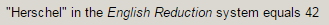Herschel discovered Uranus when he was at the age of 42 on a date with 33 numerology
3/13/1781 - 3+13+1+7+8+1 = 33
3/13/1781 - 3+1+3+1+7+8+1 = 24 (reflection of 42)

He found Uranus on 119th day after his birthday (All seeing eye - 119, High Priest - 119, Foundation - 119, Milky Way - 119..)Later in 1787 he found 2 Moons of Uranus that were named Oberon and TitaniaThey were discovered on 1/11/1787 - 1+1+1+1+7+8+7 = 26 (God = 26, Mason = 26)
1/11/87 - 1+11+87 = 99 (Thirteen = 45/99, Hershel was born on 11/15/1738 - 11+15+1+7+3+8 = 45, his initials are WH - 13)Oberon's alternative name is "Uranus IV" = 3+9+1+5+3+1+9+4 = 35 (1/11/1787 - 1+11+1+7+8+7 = 35)
Titania - "Uranus III" = 3+9+1+5+3+10 + 9+9+9 = 58 (S exception)

He found Moons of Uranus on 58th day after his birthday (Science = 58, Gregorian = 58, Calendar = 58, Freemasonry = 58, Banking = 58)Those discoveries were made from his "Observatory house" from where he found 2 Moons of Saturn as well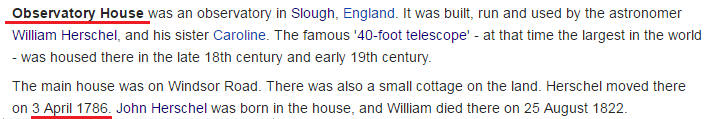Observatory House = 6+2+10+5+9+4+1+2+6+9+7 + 8+6+3+10+5 = 93 (S exception)
Observatory House = 6+2+10+5+9+22+1+2+6+9 + 8+6+3+10+5 = 111, S and V exceptions (Moons of Uranus were discovered on 1/11)

He moved there on April Third in 1786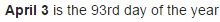4/3/86 - 4+3+86 = 93 (Saturn = 93)

There is 1 more reason April 3rd is special besides being 93rd day, it is the only date in the year that has 511 English Gematria, 511 converted to Octal numbering system is 777, and 77 converted to Octal is 115, which is extremely interesting as well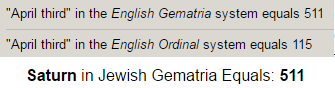Also Titania and Oberon were discovered on 314th day after he moved in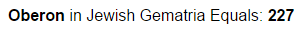227 - 22/7 = 3.14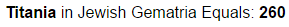Earlier I mentioned how they were found on date with 26 numerology

Now for the Moons of Saturn that he discovered in 1789

First he discovered Enceladus (Saturn II) on 8/28/1789

Enceladus = 5+5+3+5+3+1+4+3+10 - 39 (S exception) - reflection of 93
Saturn II = 1+1+2+3+9+5+9+9 = 39

8/28/1789 - 8+2+8+1+7+8+9 = 43
8/28/1789 - 8+28+17+89 = 142He discovered Enceladus 21 days before discovering next Moon of Saturn (Mimas)Orbital period of Enceladus is perfect as you can imagineAlso as expected the second Moon was discovered on a date with 42 numerology,  it is one of the most hilarious looking Moons, very much resembling Death Star from Star Wars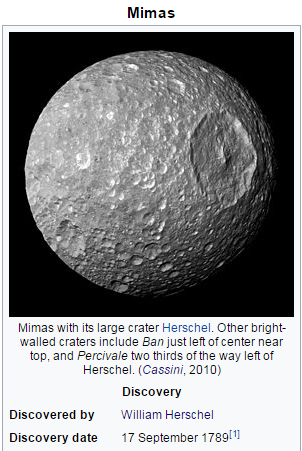9/17/1789 - 9+1+7+1+7+8+9 = 42 (Freemason = 42, Zionism = 42, Outer Space = 42, Big Bang = 42)
9/17/1789 - 9+17+1+7+8+9 = 51
9/17/89 - 9+17+89 = 115 (Freemasons = 115, Scottish Rite Freemasonry = 115 E. Reduction)

Here are some stats on Mimas from NASA's page - http://solarsystem.nasa.gov/planets/mimas/facts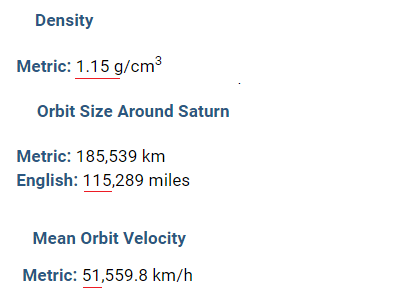Also he discovered Mimas 59 days before his 51st birthday

Mimas = 13+9+13+1+19 = 55 (Heaven = 55, Sky = 55, Satan = 55)Saturn I = 10+1+2+3+9+5+9 = 39 (S exception)

Herschel was the First president of the Royal Astronomical Society and his son John Herschel served 3 terms as the president3/10/1820 - 3+10+18+20 = 51 (Conspiracy = 51)
3/10/20 - 33

He died in in 'Slough'Observatory house is located at 51° North

He was buried at "Saint Laurence's Church" = 105 (S exceptions)Died on - 8/25/1822 - 8+25+18+22 = 73 (21st prime number)
8/25 = 8+25= 33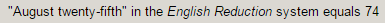There's few more stuff I could go into but I've already said enough

1.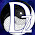1.